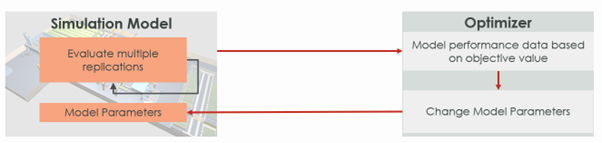EN

# What is Simulation-based optimization and when it is needed?

| June 7, 2023 |

A simulation model is a virtual representation of the real system. Simulation modeling is a powerful technique for solving complex business problems. The objective of the model is always to support decision-making. We are trying to answer questions such as: what the best action is, what is the ideal number of operators, what is the best layout, what is the ideal inventory level, etc. We are prescribing the best action and so inherently we are trying to solve an optimization problem using simulation.

There are various optimization techniques such as analytical optimization, linear programming, mixed integer programming, dynamic programming, constraint programming as well as heuristic and meta-heuristic optimization. These optimization techniques are quite useful and powerful. Depending on the problem, a better-suited technique may be chosen to find the optimal solution. However, real-world problems are complex. They can have multiple feedback loops, non-linear interactions, inherent process variation, and disruptions. The nature of the problem may be NP-hard. In such cases, sometimes it is possible to linearize the problem by taking some assumptions. In some special cases, it may be possible to make an NP-hard problem tractable by using methods like column generation and benders decomposition. But a wide variety of problems may still be out of reach for pure optimization methods. This is exactly where a simulation-based optimization approach should be applied.

### Best of both worlds

Most Simulation tools such as AnyLogic, Simio, FlexSim, etc. have a built-in optimizer called OptQuest, and some other tools allow integration with any third-party optimizers. OptQuest optimizer is based on a metaheuristic. The image below shows a high-level overview of the simulation-based optimization approach. The simulation model captures the relevant details of the real system. The parameters or decision variables are exposed to the optimizer.

In the optimization experiment, we setup the objective value function and set either a minimize or maximize objective. For each decision variable, we define the range in which the optimizer will search for an optimal solution and additionally, we may define a step size. We can also define any constraints on the decision variables. When we run this optimization experiment the optimizer changes the value of the decision variables (each such trial is called an iteration) and observes the objective value. For each iteration, the simulation runs multiple times with the same set of values for the decision variables. Each of these runs is called replications and they are necessary to incorporate the inherent stochastic nature of the system. The number of such replications can be defined by the user in the optimization settings. The optimizer will infer how good or bad an iteration is based on the average value of the objective function across these replications. The optimizer runs multiple such iterations and uses a metaheuristic strategy to arrive at the best solution.We can specify a fixed number of iterations after which the optimizer will stop, or we can allow the optimizer to run till it finds no improvement in the past many iterations.

### No free lunch?

Since this simulation-based optimization approach uses a metaheuristic optimizer it is worth mentioning that researchers in the field of metaheuristics suggest that as the problem gets larger, the optimizer performance degrades. To increase performance, we must design the metaheuristic in a more problem-specific way. The OptQuest optimizer is essentially a black-box optimizer. The “No Free Lunch Theorem” in optimization states that: The design of a general black-box optimization method is not possible. However, in practice, we have solved multiple real-world problems using the simulation-based optimization approach.